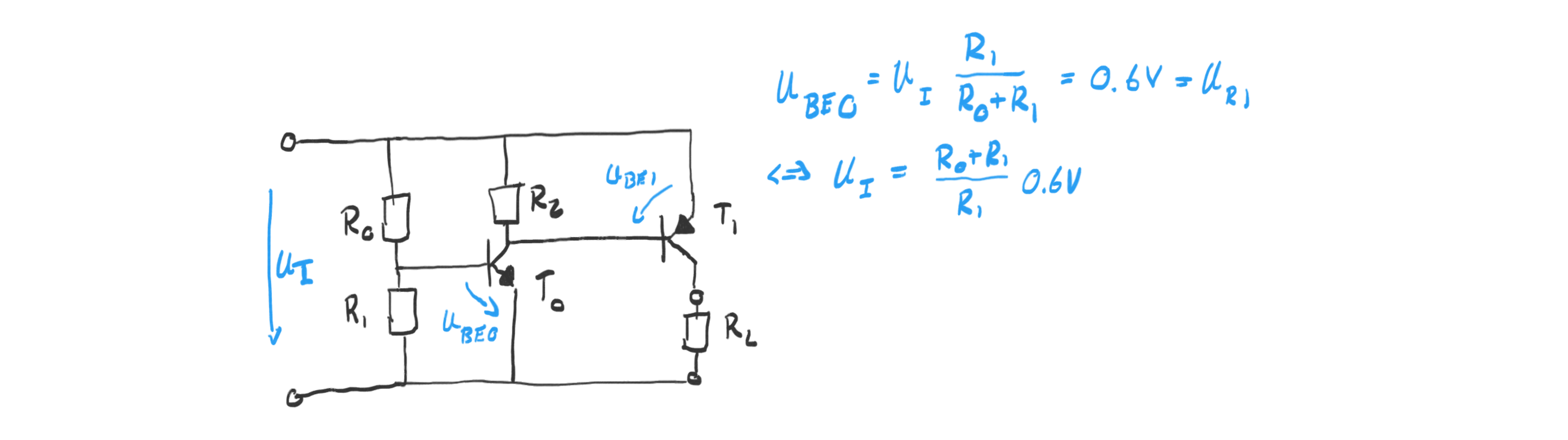# Julian Straub

## creations and thoughts

Solar cells are non-ideal power sources that need managing in order to derive maximum power from them. The one of the reasons are the non-negligible series and parallel resistance in solar cells. The I-U and I-P plot of a solar cell shown below illustrates the point: we have to regulate the voltage or current drawn from the solar cell in order to reach a point of high power output. The maximum is called maximum power point (MPP).Maximum power point trackers (MPPT) are a complex topic in themselves and important for high-power solar cell modules where efficiency is important. For smaller applications of for example charging a battery from a small module adafruit argues that ensuring a fixed solar-cell voltage is good enough. In their design of a solar-cell battery charger, they ensure that the solar cell voltage stays at about $$0.45V$$ per cell via the charging IC directly.

I was wondering how to stabilize the solar cell voltage close to the MPP in the simplest way for the use in light harvesters. The analog solar cell voltage stabilizer depicted in the circuit below regulates the output current such that the input voltage $$U_I$$ stays at a fixed voltage programmed via the voltage divider. This lets us then choose an input voltage close to the MPP of the solar cell.Specifically, the voltage $$U_{R1}$$ over resistor $$R_1$$ is held fixed at the base emitter voltage of $$T_0$$ of roughly $$0.6V$$ .

We can examine the workings of the regulator by imagining two states: (1) the input voltage is lower than the desired voltage and (2) the input voltage is higher. In case (1), the voltage over $$R_1$$ is lower than $$0.6V$$ closing transistor $$T_0$$ . This leads to a decrease of the voltage over the pnp transistor $$T_1$$ bases emitter voltage because of the pull-up resistor $$R_2$$ . $$T_1$$ also starts closing and hence reducing the flow of current $$I_o$$ into the load. The decreasing demand on current from the solar cell increases its voltage output according to the operating characteristics of a solar cell shown in the I-U plot in the beginning.

In the case (2) of higher-than-desired input voltage, the behavior of the regulator is the inverse: the output current is increased which leads to a decrease of the input voltage. The voltage over $$R_1$$ and hence $$U_{BE0}$$ of npn transistor $$T_0$$ is greater than $$0.6V$$ and hence opens $$T_0$$ completely. This pulls down the base of pnp transistor $$T_1$$ so that $$T_1$$ also goes into saturation and hence increases the current flow into the load.

Note, that the analog input voltage stabilizer is low drop out (LDO): when the output transistor $$T_1$$ is fully open the minimum voltage drop and hence “wasted” energy of the stabilizer is only the collector-emitter voltage of the pnp transistor during saturation which is usually given as less than $$0.2V$$ .

From published work (see above) and an article from adafruit it seems like the maximum power point per solar cell is between $$0.5V$$ and $$0.45V$$ . This means for a $$6V$$ solar cell array (of 10 cells) the maximum power point is between $$4.5V$$ and $$5$$ . We can set this input voltage using the analog voltage stabilizer by the following choice of parts:

• $$R_0=7.5k\Omega$$ , $$R_1=47k\Omega$$ , $$R_2=10k\Omega$$
• $$T_0$$ is a 2N3904 npn, $$T_1$$ is a 2N3906 pnp transistor